# Temperature Coefficient of Resistance (Formula And Examples)

Contents

As we discussed on the page under title resistance variation with the temperature, the electrical resistance of every substance changes with a change in its temperature.

## What is the Temperature Coefficient of Resistance?

The temperature coefficient of resistance measures changes in the electrical resistance of any substance per degree of temperature change.

Let us take a conductor having a resistance of R0 at 0oC and Rt at toC, respectively.
From the equation of resistance variation with temperature, we get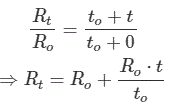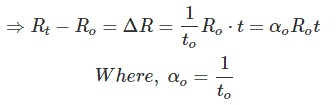This αo is called the temperature coefficient of resistance of that substance at 0oC.
From the above equation, it is clear that the change in electrical resistance of any substance due to temperature mainly depends upon three factors –

1. the value of resistance at the initial temperature,
2. the rise of temperature and
3. the temperature coefficient of resistance αo.This αo is different for different materials, so different temperatures are different in different materials.

So the temperature coefficient of resistance at 0oC of any substance is the reciprocal of that substance’s inferred zero resistance temperature.

So far, we have discussed the materials that resistance increases with an increase in temperature. Still, there are many materials whose electrical resistance of which decreases with a decrease in temperature.

Actually, in metal, if the temperature increases, the random motion of free electrons and interatomic vibration inside the metal increase, which result in more collisions.

More collisions resist the smooth flow of electrons through the metal; hence the resistance of the metal increases with the temperature rise. So, we consider the temperature coefficient of resistance as positive for metal.

But in semiconductors or other non-metal, the number of free electrons increases with an increase in temperature.

Because at a higher temperature, due to sufficient heat energy supplied to the crystal, a significant number of covalent bonds get broken, and hence more free electrons get created.

That means if temperature increases, a significant number of electrons comes to the conduction bands from valence bands by crossing the forbidden energy gap.

As the number of free electrons increases, the resistance of this type of non-metallic substance decreases with an increase in temperature. Hence temperature coefficient of resistance is negative for non-metallic substances and semiconductors.

If there is approximately no change in resistance with temperature, we can consider the value of this coefficient as zero. The alloy of constantan and manganin has a temperature coefficient of resistance of nearly zero.

The value of this coefficient is not constant; it depends on the initial temperature on which the increment of resistance is based.

When the increment is based on an initial temperature of 0oC, the value of this coefficient is αo – which is nothing but the reciprocal of the respective inferred zero resistance temperature of the substance.

But at any other temperature, the temperature coefficient of electrical resistance is not the same as this αo. Actually, for any material, the value of this coefficient is maximum at 0oC temperature.

Say the value of this coefficient of any material at any toC is αt, then its value can be determined by the following equation,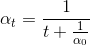The value of this coefficient at a temperature of t2oC in the term of the same at t1oC is given as,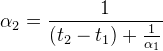### Review the Concept of Temperature Coefficient of Resistance

The electrical resistance of conductors such as silver, copper, gold, aluminum, etc., depends on electrons’ collision process within the material.

As the temperature increases, this electron collision process becomes faster, which results in increased resistance with the rise in the temperature of the conductor. The resistance of conductors generally rises with rising in temperature.

If a conductor has R1 resistance at t1oC and rises the temperature, its resistance becomes R2 at t2oC.

This rise in resistance (R2 – R1) with rising in temperature (t2 – t1) depends on the following things –By combining the above effects,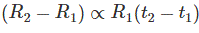Where α is the temperature coefficient of resistance of material at t1oC.

From Equation (1)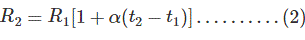If at a particular temperature, we know the resistance and temperature coefficient of resistance of the material, we can find out the resistance of material at other temperatures by using equation (2).

The Temperature Coefficient of Resistance of some Materials or Substances

The temperature coefficient of resistance of some materials/substances at 20oC are listed below-

 Sl. No. Material/Substances Chemical Symbol/Chemical composition Temperature coefficient of resistance /oC (at 20oC) 1 Silver Ag 0.0038 2 Copper Cu 0.00386 3 Gold Au 0.0034 4 Aluminum Al 0.00429 5 Tungsten W 0.0045 6 Iron Fe 0.00651 7 Platinum Pt 0.003927 8 Manganin Cu = 84% + Mn = 12% + Ni = 4% 0.000002 9 Mercury Hg 0.0009 10 Nichrome Ni = 60% + Cr = 15% + Fe = 25% 0.0004 11 Constantan Cu = 55% + Ni = 45% 0.00003 12 Carbon C – 0.0005 13 Germanium Ge – 0.05 14 Silicon Si – 0.07 15 Brass Cu = 50 – 65% + Zn = 50 – 35% 0.0015 16 Nickel Ni 0.00641 17 Tin Sn 0.0042 18 Zinc Zn 0.0037 19 Manganese Mn 0.00001 20 Tantalum Ta 0.0033

Effect of Temperature on Temperature Coefficient of Resistance of a Material

The temperature coefficient of resistance of material also changes with temperature.

If αo is the temperature coefficient of resistance of material at 0oC, then from equation (2), the resistance of material at toC,Where R0 is the Resistance of material at 0oC

Similarly, if the temperature coefficient of resistance of material at toC is αt, then the resistance of the material at 0oC, from equation (2)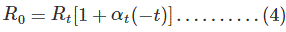Where, Rt is the Resistance of material at to C

From equation (3) and (4)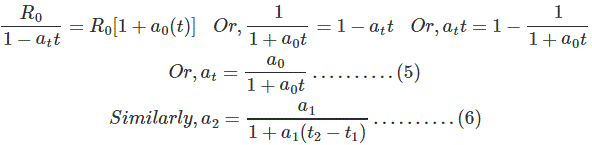Where, α1and α2 the temperature coefficient of resistance of material at t1oC and t2oC, respectively.

Hence, if we know the temperature coefficient of resistance of a material at a particular temperature, we may find out the temperature coefficient of material at any other temperature by using equation (6).

The conducting materials have a large and positive temperature coefficient of resistance. Therefore, the resistance of conducting materials (metals) rises with the rising temperature.

The semiconductors and insulating material are having a negative temperature coefficient of resistance. Therefore, the resistance of semiconductors and insulators decreases with rising in temperature.

Alloys, such as manganin, constantan, etc., have a very low and positive temperature coefficient of resistance.

Therefore, the resistance of alloys increases with rising in temperature.

Still, this rise in resistance is very low (almost negligible) compared to other metals, making these alloys suitable for measuring instruments.

Want To Learn Faster? 🎓
Get electrical articles delivered to your inbox every week.
No credit card required—it’s 100% free.